The Rocscience International Conference 2021 Proceedings are now available. Read Now

# Sarma Non-Vertical Slice Method

To use the Sarma Non-Vertical Slice limit equilibrium analysis method:

1. Select Project Settings > Methods > Sarma Non-Vertical Slices
2. Enter the analysis parameters described below.

NOTE: the Sarma Non-Vertical Slice analysis method cannot be run simultaneously with the vertical slice analysis methods.

Sarma (1979) developed a limit equilibrium analysis method for non-vertical slices, where the angle of each slice is found as part of the solution. In this method, the critical acceleration factor Kc is calculated, with the assumption that under the influence of the force (KcWi) the factor of safety on the slip surface is equal to one.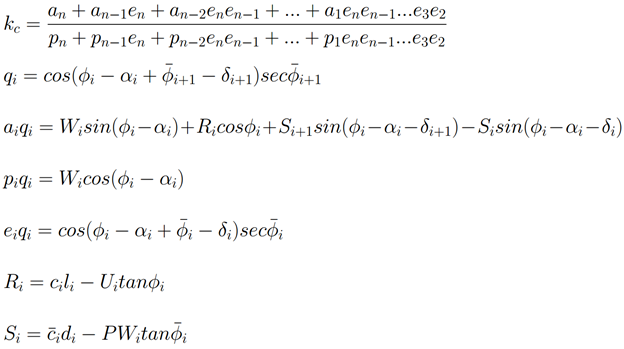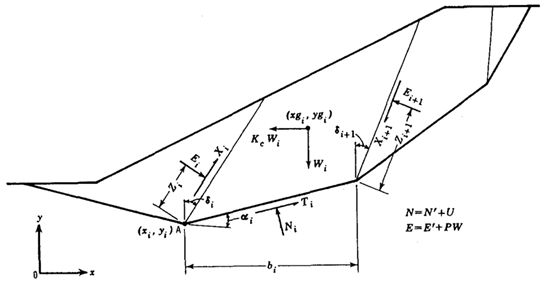Where the symbols are defined as:

•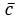is the average cohesion on the side of the slice
•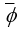is the average friction angle on the side of the slice
• a is the angle of the base of the slice (relative to the horizontal axis)
• d is the angle of the side of the slice (relative to the vertical axis)
• W is the weight of the slice
• l is the length of the base of the slice
• d is the length of the side of the slice (from slip surface to ground surface)
• U is the force due to pore water pressure on base of slice
• PW is the force due to pore water pressure on the side of the slice

The factor of safety (Fs) is then calculated through an iterative process where the strength parameters are reduced until a critical acceleration of zero is reached: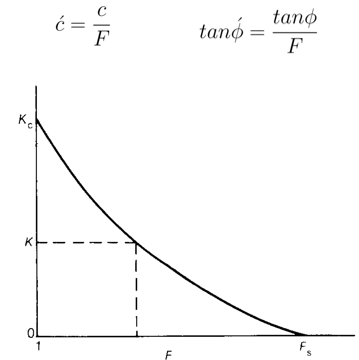### NUMBER OF SLICES

The Number of Slices is the number of non-vertical slices (wedges) into which the sliding mass for each slip surface will be divided. The default number of 25 slices is sufficient to obtain an accurate solution for most problems.

Slice boundaries will be located at each vertex of a failure surface. If the Add slices at material intersections checkbox is selected, slice boundaries will also be located at these intersection points. Additional slice boundaries will be inserted as required to achieve the specified number of slices.

### TOLERANCE

The Tolerance is the difference in safety factor between two successive iterations of the limit equilibrium analysis procedure, at which the solution is considered to have converged, and the iteration process is stopped. For Sarma Non-Vertical Slice analysis the default value of 0.0001 is recommended.

NOTE: the Sarma Non-Vertical Slice method requires a smaller tolerance value than the Vertical Slice methods in order to achieve an accurate solution.

### MAXIMUM NUMBER OF ITERATIONS

The Maximum Iterations is the maximum number of iterations allowed in the limit equilibrium analysis, for each slip surface. The default value is 75 which is sufficient for most analyses.

### SLICE ANGLES

The factor of safety depends on the set of values of slice boundary angles (δ). The objective is to find the critical set, which is defined as the set of angles that results in the lowest factor of safety. The following options are available for defining the Sarma Non-Vertical slice angles:

• Optimized (Global Minimum or All Surfaces)
• User Defined (Bisection, Weighted Average Normal, Vertical)

See the Sarma Slice Angles topic for details.

### SARMA INTERSLICE STRENGTH OPTIONS

The factor of safety is also dependant on the cohesion and friction angle along the interslice boundaries. The following options are available for defining the interslice shear strength for the Sarma analysis method.

• Computed Average Value
• User Defined (cohesion, friction angle)

See the Sarma Interslice Strength Options topic for details.

### ADD SLICES AT MATERIAL INTERSECTIONS

If this checkbox is selected, slice boundaries will automatically be created at the intersections of material boundaries and slip surfaces. This ensures that the base of each slice will have a single material type.

If this checkbox is off, then slice locations will ignore material / slip surface intersections, which may lead to multiple material types on the base of a single slice. Since the material type assigned to a slice base is determined only from the material at the CENTER POINT of the slice base, this will lead to inaccurate strength properties for slices with multiple materials along the base.

By default this option is turned ON. It should not be turned OFF unless you wish to compute results using the above assumption.

### USAGE

Active-passive wedge failures such as those which occur in spoil piles on sloping foundations or in clay core dam embankments can be analyzed using this method. In a model with anisotropic material, unlike other limit equilibrium methods, the factor of safety is affected when the slices are aligned with the direction of the weak layers.

The Sarma method is intended primarily for non-circular slip surfaces. It can be used for circular slip surfaces, however for rotational (circular) failure, the advantages of considering a wedge type internal failure mechanism are minimized due to the nature of the rotational mechanism.

### LIMITATIONS

Currently Excess Pore Pressure and Rapid Drawdown Analyses cannot be performed using Sarma non-vertical method. In addition, the line of thrust cannot be drawn for Sarma non-vertical.

### VERIFICATION

See the Slope Stability Verification (Sarma Method) document for verification examples of models solved using the Sarma non-vertical slice method.